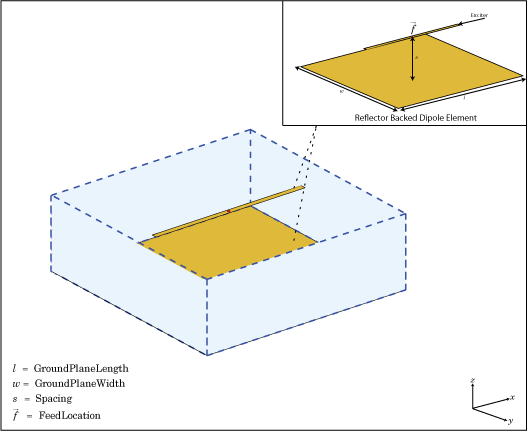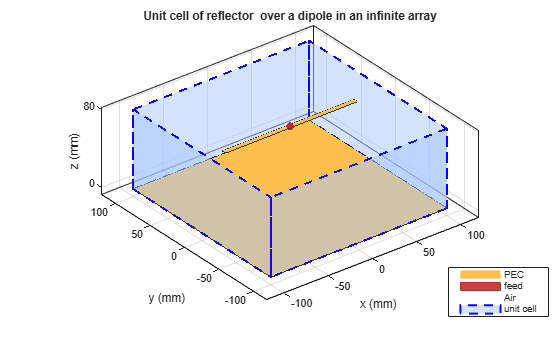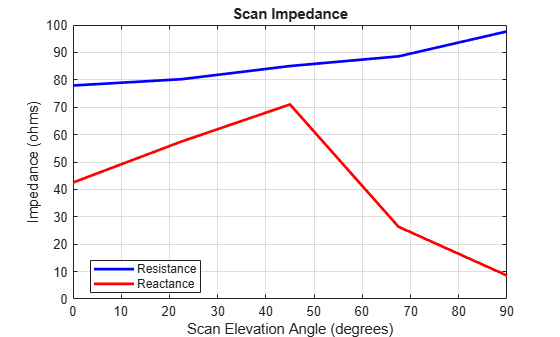Documentation

# infiniteArray

Create 2-D custom mesh antenna on X-Y plane

## Description

The `infiniteArray` object is an infinite antenna array in the X-Y plane. Infinite array models a single antenna element called the unit cell. Ground plane of the antennas specifies the boundaries of the unit cell. Antennas without a ground plane require a reflector. By default, the infinite array has reflected-backed dipoles as antenna elements. The default dimensions are chosen for an operating frequency of 1 GHz.## Creation

### Description

``` `infa = infiniteArray` creates an infinite antenna array in the X-Y plane. ```

example

``` `infa = infiniteArray(Name,Value)` creates an infinite antenna array with additional properties specified by one, or more name-value pair arguments. `Name` is the property name and `Value` is the corresponding value. You can specify several name-value pair arguments in any order as `Name1, Value1, ..., NameN, ValueN`. Properties not specified retain default values.```

## Properties

expand all

Type of individual antenna elements in unit cell, specified as an object. Antenna without a groundplane is backed using a reflector. The ground plane size specifies the unit cell boundaries.

Example: `'Element'`,`reflector`

Scan direction in azimuth plane, specified as a scalar in degrees.

Example: `'ScanAzimuth'`,`25`

Data Types: `double`

Scan direction in elevation plane, specified as a scalar in degrees.

Example: `'ScanElevation'`,`80`

Data Types: `double`

## Object Functions

 `numSummationTerms` Change number of summation terms for calculating periodic Green's function

## Examples

collapse all

Create an infinite array with reflector-backed dipoles as unit cells. Scan the array at boresight. Visualize the unit cell.

```infa = infiniteArray('Element',reflector,'ScanAzimuth',0, ... 'ScanElevation',90); show(infa)```Calculate the scan impedance of an infinite array at 1GHz. To calculate the impedance, scan the infinite array from boresight to horizon in the elevation plane.

```infa = infiniteArray; theta0deg = linspace(0,90,5); zscan = nan(1,numel(theta0deg)); for j = 1:numel(theta0deg) infa.ScanElevation = theta0deg(j); zscan(1,j) = impedance(infa,1e9); end plot(zscan)```Balanis, C.A. Antenna Theory: Analysis and Design. 3rd Ed. New York: Wiley, 2005.